# Changes in Energy

## Calculate Elastic Store of Energy

In order to calculate the amount of energy in the elastic store, we use this equation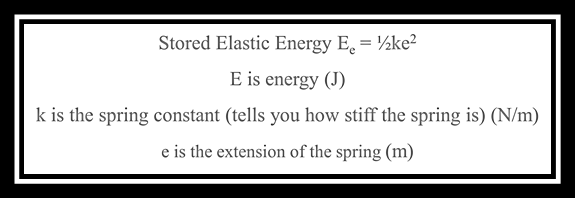The spring constant is a quantity that we meet later on in the GCSE and will be explained further in the Forces topic.

## Calculate Gravitational Store of Energy

In order to calculate energy in the gravitational store, we use this equation: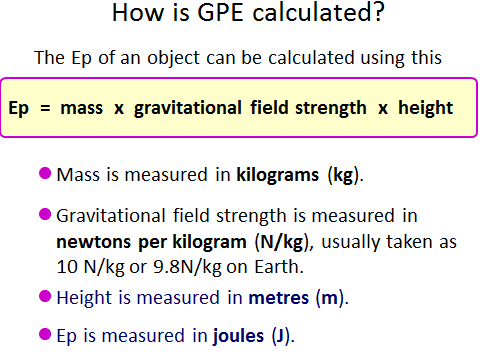This can also be written as Ep = m g h

Remember that you will be given the value for the gravitational field strength in the question (unless you are asked to calculate it!).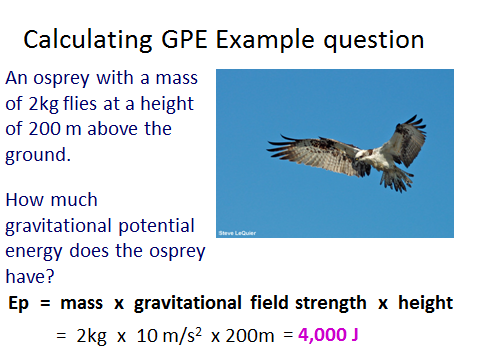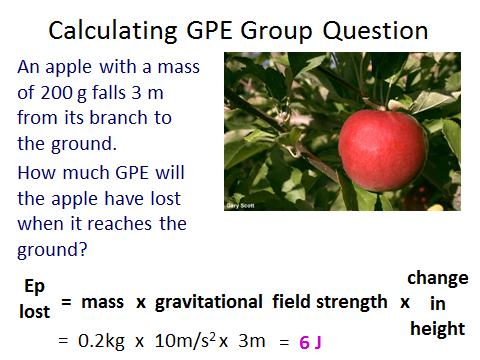Notice that the 200g is changed into 0.2kg. It is really important to ensure that kg is always used. In order to convert grams to kilograms, just divide the number by 1000.

## Calculatre Kinetic Store of Energy

In order to calculate energy in the kinetic store, we use this equation: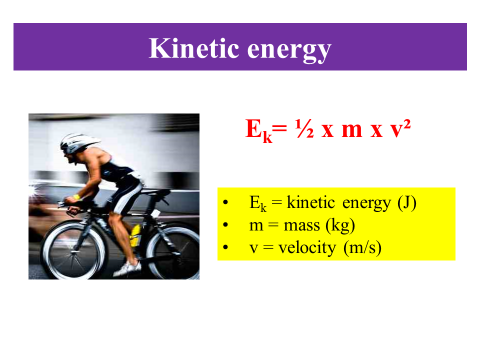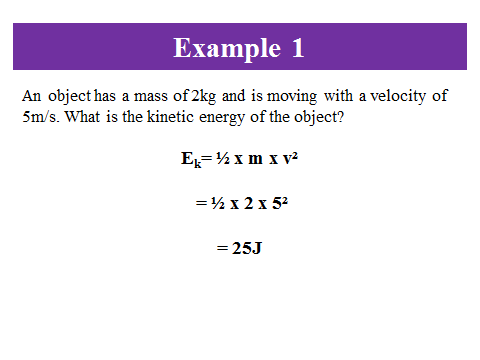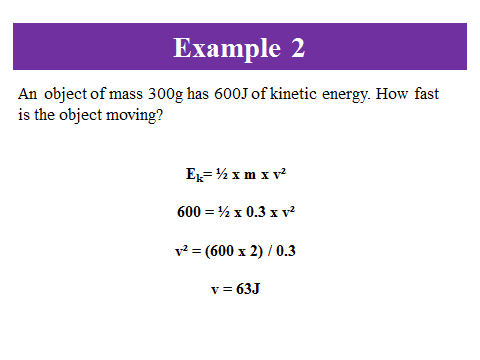## 5Mark Questions

On each paper there is one five mark equation question. It involves using two equations!

What happens is that you will need to use one equation and then use the answer generated in the second equation.

Let’s do an example with energy!

Question:

Calculate the velocity of a 5kg object which was dropped from a height of 10m.

g = 10N/kg

Working:

The first step is to see that we need to find out we are missing. To calculate velocity, the only equation we know is Ek= ½ x m x v².

We need to find v and have been given m; so we are missing Ek.

The question also gives us the height and a value for g. The only equation that suits this is Ep=mgh. We should remember that the kinetic energy at the bottom is the same value as the gravitational energy at the top.

Therefore:

Ep= 5 x 10 x 10 = 500J

So:

500 = ½ x m x v²

v² = (500 x 2) / 10 = 100

Remember to square root both sides so:

v = 10m/s

What is the kinetic energy of a 3kg ball that is rolling at 2m/s?